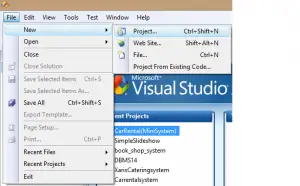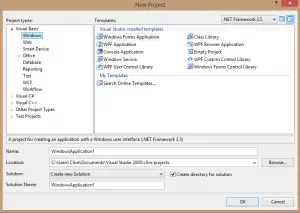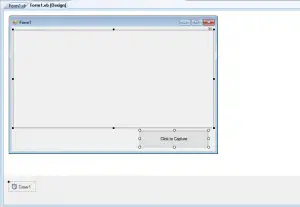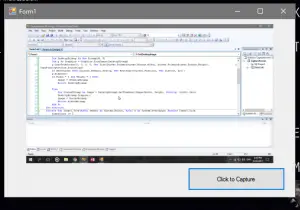How to Capture Full Screen using VB.Net

0
334

This Tutorial is all about How to Capture Full Screen using VB.Net. In this tutorial you will be able to Capture Full Screen using VB.Net So lets get Started:

• First is open the Visual Basic, Select File on the menu, then click New and create a new project.• Then a New Project Dialog will appear. You can rename your project, depending on what you like to name it. After that click OK• After that, design your form like this just like what I’ve shown you below.
Add a Picturebox, Button and a Timer.
Change the PictureBox SizeMode property to Zoom.
Change the Timer Enabled property to True and change Interval to 1000.• Then add this following Declarations.
[vbnet] Public Shared Image As Image
Public Shared Bounds As Rectangle
Public Shared TimerCount As Integer
[/vbnet]
• Also add this following Functions.
[vbnet] Shared Function GetDesktopImage(Optional ByVal Width As Integer = 0, Optional ByVal Height As Integer = 0, Optional ByVal ShowCursor As Boolean = True) As Image
Dim W As Integer = Screen.PrimaryScreen.Bounds.Width
Dim H As Integer = Screen.PrimaryScreen.Bounds.Height
Dim DesktopBitmap As New Bitmap(W, H)
Dim g As Graphics = Graphics.FromImage(DesktopBitmap)
g.CopyFromScreen(0, 0, 0, 0, New Size(Screen.PrimaryScreen.Bounds.Width, Screen.PrimaryScreen.Bounds.Height), CopyPixelOperation.SourceCopy)
If ShowCursor Then Cursors.Default.Draw(g, New Rectangle(Cursor.Position, New Size(32, 32)))
g.Dispose()
If Width = 0 And Height = 0 Then
Image = DesktopBitmap
Return DesktopBitmapElse
Dim ScaledBitmap As Image = DesktopBitmap.GetThumbnailImage(Width, Height, Nothing, IntPtr.Zero)
DesktopBitmap.Dispose()
Image = ScaledBitmap
Return ScaledBitmap
End If
End Function

[/vbnet]
• Add this code to the timer.
[vbnet] Private Sub Timer1_Tick(ByVal sender As System.Object, ByVal e As System.EventArgs) Handles Timer1.Tick
TimerCount += 1If TimerCount = 1 And Me.WindowState = FormWindowState.Minimized Then
PictureBox1.Image = GetDesktopImage()

End If
If TimerCount = 2 And Me.WindowState = FormWindowState.Minimized Then
Me.WindowState = FormWindowState.Normal
End If
End Sub

[/vbnet]
• Add this code to the button.
[vbnet] Private Sub Button1_Click(ByVal sender As System.Object, ByVal e As System.EventArgs) Handles Button1.Click
Me.WindowState = FormWindowState.Minimized
TimerCount = 0
End Sub
[/vbnet]
• Finally, Click F5 to run the Program.

Output: